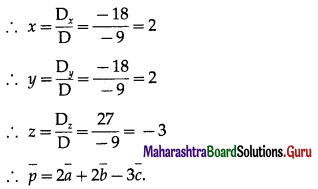# Maharashtra Board 12th Maths Solutions Chapter 5 Vectors Ex 5.1

Balbharti 12th Maharashtra State Board Maths Solutions Book Pdf Chapter 5 Vectors Ex 5.1 Questions and Answers.

## Maharashtra State Board 12th Maths Solutions Chapter 5 Vectors Ex 5.1

Question 1.
The vector $$\bar{a}$$ is directed due north and $$|\bar{a}|$$ = 24. The vector $$\bar{b}$$ is directed due west and $$|\bar{b}|$$ = 7. Find $$|\bar{a}+\bar{b}|$$.
Solution: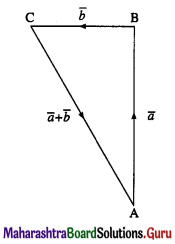Let $$\overline{\mathrm{AB}}$$ = $$\bar{a}$$, $$\overline{\mathrm{BC}}$$ = $$\bar{b}$$
Then $$\overline{\mathrm{AC}}$$ = $$\overline{\mathrm{AB}}$$ + $$\overline{\mathrm{BC}}$$ = a + b
Given : $$|\bar{a}|$$ = $$|\overline{\mathrm{AB}}|$$ = l(AB) = 24 and
$$|\bar{b}|$$ = $$|\overline{\mathrm{BC}}|$$ = l(BC) = 7
∴ ∠ABC = 90°
∴ [l(AC)]2 = [l(AB)]2 + [l(BC)]2
= (24)2 + (7)2 = 625
∴ l(AC) = 25 ∴ $$|\overline{\mathrm{AC}}|$$ = 25
∴ $$|\bar{a}+\bar{b}|$$ = $$|\overline{\mathrm{AC}}|$$ = 25.

Question 2.
In the triangle PQR, $$\overline{\mathrm{PQ}}$$ = 2$$\bar{a}$$ and $$\overline{\mathrm{QR}}$$ = 2$$\bar{b}$$. The mid-point of PR is M. Find following vectors in terms of $$\bar{a}$$ and $$\bar{b}$$.
(i) $$\overline{\mathrm{PR}}$$
Solution: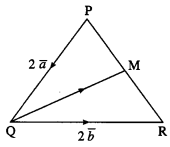Given : $$\overline{\mathrm{PQ}}$$ = 2$$\bar{a}$$, $$\overline{\mathrm{QR}}$$ = 2$$\bar{b}$$
(i) $$\overline{\mathrm{PR}}$$ = $$\overline{\mathrm{PQ}}$$ + $$\overline{\mathrm{QR}}$$
= 2$$\bar{a}$$ + 2$$\bar{a}$$.(ii) $$\overline{\mathrm{PM}}$$
Solution:
∵ M is the midpoint of PR
∴ $$\overline{\mathrm{PM}}$$ = $$\frac{1}{2} \overline{\mathrm{PR}}$$ = $$\frac{1}{2}$$[2$$\bar{a}$$ + 2$$\bar{b}$$]
= $$\bar{a}$$ + $$\bar{b}$$.

(iii) $$\overline{\mathrm{QM}}$$
Solution:
$$\overline{\mathrm{RM}}$$ = $$\frac{1}{2}(\overline{\mathrm{RP}})$$ = $$-\frac{1}{2} \overline{\mathrm{PR}}$$ = $$-\frac{1}{2}$$(2$$\bar{a}$$ + 2$$\bar{b}$$)
= –$$\bar{a}$$ – $$\bar{b}$$
∴ $$\overline{\mathrm{QM}}$$ = $$\overline{\mathrm{QR}}$$ + $$\overline{\mathrm{RM}}$$
= 2$$\bar{b}$$ – $$\bar{a}$$ – $$\bar{b}$$
= $$\bar{b}$$ – $$\bar{a}$$.

Question 3.
OABCDE is a regular hexagon. The points A and B have position vectors $$\bar{a}$$ and $$\bar{b}$$ respectively, referred to the origin O. Find, in terms of $$\bar{a}$$ and $$\bar{b}$$ the position vectors of C, D and E.
Solution: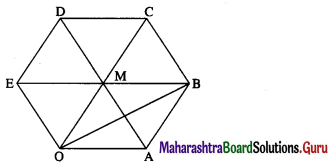Given : $$\overline{\mathrm{OA}}$$ = $$\bar{a}$$, $$\overline{\mathrm{OB}}$$ = $$\bar{a}$$ Let AD, BE, OC meet at M.
Then M bisects AD, BE, OC.
$$\overline{\mathrm{AB}}$$ = $$\overline{\mathrm{AO}}$$ + $$\overline{\mathrm{OB}}$$ = –$$\overline{\mathrm{OA}}$$ + $$\overline{\mathrm{OB}}$$ = –$$\bar{a}$$ + $$\bar{b}$$ = $$\bar{b}$$ – $$\bar{a}$$
∵ OABM is a parallelogram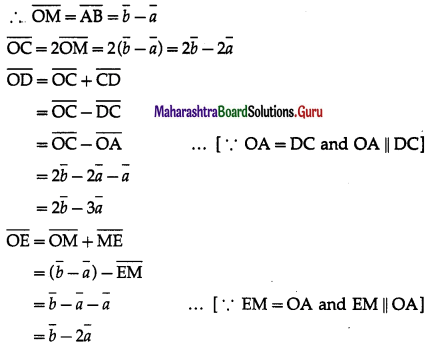Hence, the position vectors of C, D and E are 2$$\bar{b}$$ – 2$$\bar{a}$$, 2$$\bar{b}$$ – 3$$\bar{a}$$ and $$\bar{b}$$ – 2$$\bar{a}$$ respectively.Question 4.
If ABCDEF is a regular hexagon, show that $$\overline{\mathrm{AB}}$$ + $$\overline{\mathrm{AC}}$$ + $$\overline{\mathrm{AD}}$$ + $$\overline{\mathrm{AE}}$$ + $$\overline{\mathrm{AF}}$$ = 6$$\overline{\mathrm{AO}}$$, where O is the center of the hexagon.
Solution: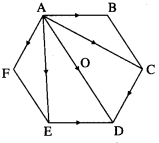ABCDEF is a regular hexagon.
∴ $$\overline{\mathrm{AB}}$$ = $$\overline{\mathrm{ED}}$$ and $$\overline{\mathrm{AF}}$$ = $$\overline{\mathrm{CD}}$$
∴ by the triangle law of addition of vectors,Question 5.
Check whether the vectors $$2 \hat{i}+2 \hat{j}+3 \hat{k}$$, + $$-3 \hat{i}+3 \hat{j}+2 \hat{k}$$, + $$3 \hat{i}+4 \hat{k}$$ form a triangle or not.
Solution:
Let, if possible, the three vectors form a triangle ABC
with $$\overline{A B}$$ = $$2 \hat{i}+2 \hat{j}+3 \hat{k}$$, $$\overline{B C}$$ = $$3 \hat{i}+3 \hat{j}+2 \hat{k}$$, $$\overline{A C}$$ = $$3 \hat{i}+4 \hat{k}$$
Now, $$\overline{A B}$$ + $$\overline{B C}$$
= $$(2 \hat{i}+2 \hat{j}+3 \hat{k})$$ + $$(-3 \hat{i}+3 \hat{j}+2 \hat{k})$$
= $$-\hat{i}+5 \hat{j}+5 \hat{k} \neq 3 \hat{i}+4 \hat{k}$$ = $$\overline{\mathrm{AC}}$$
Hence, the three vectors do not form a triangle.Question 6.
In the figure 5.34 express $$\bar{c}$$ and $$\bar{d}$$ in terms of $$\bar{a}$$ and $$\bar{b}$$. Find a vector in the direction of $$\bar{a}$$ = $$\hat{i}-2 \hat{j}$$ that has magnitude 7 units.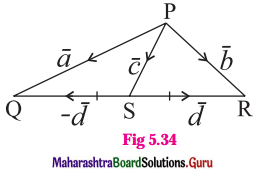Solution:
$$\overline{\mathrm{PQ}}$$ = $$\overline{\mathrm{PS}}$$ + $$\overline{\mathrm{SQ}}$$
∴ $$\bar{a}$$ = $$\bar{c}$$ – $$\bar{d}$$ … (1)
$$\overline{\mathrm{PR}}$$ = $$\overline{\mathrm{PS}}$$ + $$\overline{\mathrm{SR}}$$
∴ $$\bar{b}$$ = $$\bar{c}$$ + $$\bar{d}$$ … (2)
Adding equations (1) and (2), we get
$$\bar{a}$$ + $$\bar{b}$$ = ($$\bar{c}$$ – $$\bar{d}$$) + ($$\bar{c}$$ + $$\bar{d}$$) = 2$$\bar{c}$$Question 7.
Find the distance from (4, -2, 6) to each of the following :
(a) The XY-plane
Solution:
Let the point A be (4, -2, 6).
Then,
The distance of A from XY-plane = |z| = 6

(b) The YZ-plane
Solution:
The distance of A from YZ-plane = |x| = 4(c) The XZ-plane
Solution:
The distance of A from ZX-plane = |y| = 2

(d) The X-axis
Solution:
The distance of A from X-axis
= $$\sqrt{y^{2}+z^{2}}$$ = $$\sqrt{(-2)^{2}+6^{2}}$$ = $$\sqrt{40}$$ = $$2 \sqrt{10}$$

(e) The Y-axis
Solution:
The distance of A from Y-axis
= $$\sqrt{z^{2}+x^{2}}$$ = $$\sqrt{6^{2}+4^{2}}$$ = $$\sqrt{52}$$ = $$2 \sqrt{13}$$

(f) The Z-axis
Solution:
The distance of A from Z-axis
= $$\sqrt{x^{2}+y^{2}}$$ = $$\sqrt{4^{2}+(-2)^{2}}$$ = $$\sqrt{20}$$ = $$2 \sqrt{5}$$Question 8.
Find the coordinates of the point which is located :
(a) Three units behind the YZ-plane, four units to the right of the XZ-plane and five units above the XY-plane.
Solution:
Let the coordinates of the point be (x, y, z).
Since the point is located 3 units behind the YZ- j plane, 4 units to the right of XZ-plane and 5 units , above the XY-plane,
x = -3, y = 4 and z = 5
Hence, coordinates of the required point are (-3, 4, 5)

(b) In the YZ-plane, one unit to the right of the XZ-plane and six units above the XY-plane.
Solution:
Let the coordinates of the point be (x, y, z).
Since the point is located in the YZ plane, x = 0. Also, the point is one unit to the right of XZ-plane and six units above the XY-plane.
∴ y = 1, z = 6.
Hence, coordinates of the required point are (0, 1, 6).

Question 9.
Find the area of the triangle with vertices (1, 1, 0), (1, 0, 1) and (0, 1, 1).
Solution:
Let A = (1, 1, 0), B = (1, 0, 1), C = (0, 1, 1)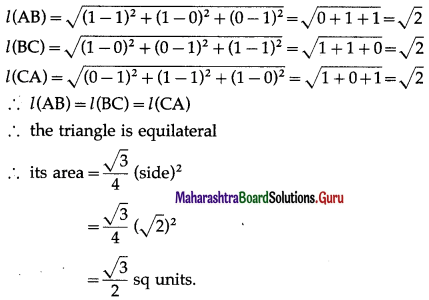Question 10.
If $$\overline{\mathrm{AB}}$$ = $$2 \hat{i}-4 \hat{j}+7 \hat{k}$$ and initial point A ≡ (1, 5, ,0). Find the terminal point B.
Solution:
Let $$\bar{a}$$ and $$\bar{b}$$ be the position vectors of A and B.
Given : A = (1, 5, 0) .’. $$\bar{a}$$ = $$\hat{i}+5 \hat{j}$$
Now, $$\overline{\mathrm{AB}}$$ = $$2 \hat{i}-4 \hat{j}+7 \hat{k}$$
∴ $$\bar{b}$$ – $$\bar{a}$$ = $$2 \hat{i}-4 \hat{j}+7 \hat{k}$$
∴ $$\bar{b}$$ = $$(2 \hat{i}-4 \hat{j}+7 \hat{k})$$ + $$\bar{a}$$
= $$(2 \hat{i}-4 \hat{j}+7 \hat{k})$$ + $$(\hat{i}+5 \hat{j})$$
= $$3 \hat{i}+\hat{j}+7 \hat{k}$$
Hence, the terminal point B = (3, 1, 7).

Question 11.
Show that the following points are collinear :
(i) A (3, 2, -4), B (9, 8, -10), C (-2, -3, 1).
Solution:
Let $$\bar{a}$$, $$\bar{b}$$, $$\bar{c}$$ be the position vectors of the points.
A = (3, 2, -4), B = (9, 8, -10) and C = (-2, -3, 1) respectively.∴ $$\overline{\mathrm{BC}}$$ is a non-zero scalar multiple of $$\overline{\mathrm{AB}}$$
∴ they are parallel to each other.
But they have the point B in common.
∴ $$\overline{\mathrm{BC}}$$ and $$\overline{\mathrm{AB}}$$ are collinear vectors.
Hence, the points A, B and C are collinear.

(ii) P (4, 5, 2), Q (3, 2, 4), R (5, 8, 0).
Solution:
Let $$\bar{a}$$, $$\bar{b}$$, $$\bar{c}$$ be the position vectors of the points.
P = (4, 5, 2), Q = (3, 2, 4), R = (5, 8, 0) respectively.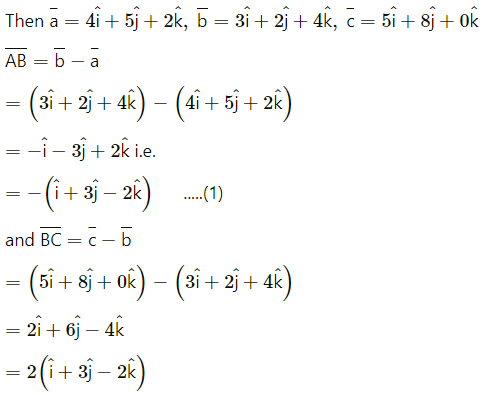= 2.$$\overline{\mathrm{AB}}$$ …[By (1)]
∴ $$\overline{\mathrm{BC}}$$ is a non-zero scalar multiple of $$\overline{\mathrm{AB}}$$
∴ they are parallel to each other.
But they have the point B in common.
∴ $$\overline{\mathrm{BC}}$$ and $$\overline{\mathrm{AB}}$$ are collinear vectors.
Hence, the points A, B and C are collinear.Question 12.
If the vectors $$2 \hat{i}-q \hat{j}+3 \hat{k}$$ and $$4 \hat{i}-5 \hat{j}+6 \hat{k}$$ are collinear, then find the value of q.
Solution:
The vectors $$2 \hat{i}-q \hat{j}+3 \hat{k}$$ and $$4 \hat{i}-5 \hat{j}+6 \hat{k}$$ are collinear
∴ the coefficients of $$\hat{i}, \hat{j}, \hat{k}$$ are proportional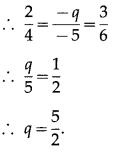Question 13.
Are the four points A(1, -1, 1), B(-1, 1, 1), C(1, 1, 1) and D(2, -3, 4) coplanar? Justify your answer.
Solution:
The position vectors $$\bar{a}$$, $$\bar{b}$$, $$\bar{c}$$, $$\bar{d}$$ of the points A, B, C, D areBy equality of vectors,
y = -2 ….(1)
2x – 2y = 2 … (2)
3y = 0 … (3)
From (1), y = -2
From (3), y = 0 This is not possible.
Hence, the points A, B, C, D are not coplanar.Question 14.
Express $$-\hat{i}-3 \hat{j}+4 \hat{k}$$ as linear combination of the vectors $$2 \hat{i}+\hat{j}-4 \hat{k}$$, $$2 \hat{i}-\hat{j}+3 \hat{k}$$ and $$3 \hat{i}+\hat{j}-2 \hat{k}$$.
Solution:By equality of vectors,
2x + 2y + 3 = -1
x – y + z = -3
-4x + 3y – 2z = 4
We have to solve these equations by using Cramer’s Rule
D = $$\left|\begin{array}{rrr} 2 & 2 & 3 \\ 1 & -1 & 1 \\ -4 & 3 & -2 \end{array}\right|$$
= 2(2 – 3) – 2(-2 + 4) + 3(3 – 4)
= -2 – 4 – 3 = -9 ≠ 0= 2(-4 + 9) – 2(4 – 12) – 1(3 – 4)
= 10 + 16 + 1 = 27